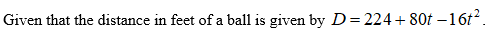# If distance in feet of a ball above ground is given by D=224+80t-16t^2 (t measured in seconds), when will the ball hit the ground? (at what value of t)?

Question
3 views

If distance in feet of a ball above ground is given by D=224+80t-16t^2 (t measured in seconds), when will the ball hit the ground? (at what value of t)?

check_circle

Step 1Step 2

When the ball hits the ground th...

### Want to see the full answer?

See Solution

#### Want to see this answer and more?

Solutions are written by subject experts who are available 24/7. Questions are typically answered within 1 hour.*

See Solution
*Response times may vary by subject and question.
Tagged in

### Other